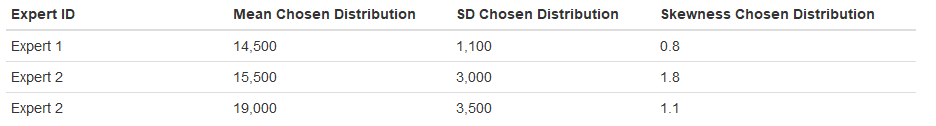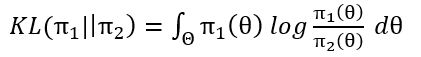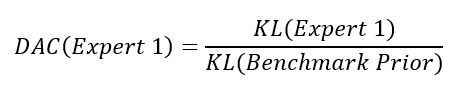# Understanding Expert Data Disagreement

## Solutions

Developed by Lion Behrens, Duco Veen and Rens van de Schoot

How to elicit expert knowledge and predictions using probability distributions

First Exercise: Elicit Expert Judgement in Five Steps

How to compare predictions of different experts using DAC values

Second Exercise: Exploring Expert Data Disagreement

⇒ Behind the scenes: Understanding the computation of DAC values

This tutorial provides the reader with a deeper understanding of how expert predictions are compared using DAC values. In the first exercise of this series, you learned how to elicit expert knowledge and predictions using probability distributions. In the second exercise of this series, it was dealt with comparing expert predictions using DAC values once the true data has been collected. In this third and last part of the series, it is aimed for a deeper understanding of how these DAC values are actually computed and what is going on behind the scenes. Therefore, an original Shiny app has been created. Open the app to conduct the exercise!

### Introduction

After having been founded as a young start-up company in 2015, the team of DataAid has successfully navigated through its first four years 2015-2018 on the market. In the former exercise of this series, three experts have (next to you) predicted the company's mean turnover per employee for the year 2018. This tutorial focuses on these three experts, whose predictions are depicted in table 1.

Table 1: Expert Predictions of Average Employee Turnover 2018### Exercise

The second exercise used DAC (= Data Agreement Criterion) values to compare the performance of the different experts. The computation of DAC values is based on Kullback-Leibler Divergences. Open the Shiny app.

The figure on the right displays the fictive scale of possible mean turnover rates per employee. The blue line represents the probability distribution that was elicited from a certain exemplary expert (“Chosen Distribution”). The red line represents the true distribution that was observed in the year 2018 (“Preferred Distribution”). The green line displays the Kullback-Leibler Divergence between the two.

In the legend on the right, the KL divergence is quantified as a single value, which expresses the area under the curve of its distribution. The smaller the number, the smaller the area under the curve, the smaller the discrepancy (divergence) between the two distributions. In other words, a small KL divergence means an accurate prediction or approximation of the preferred distribution by the chosen distribution.

a) Start by focusing on the formula in the upper left of the figure. The single KL Divergence value is defined aswhere Θ is the set of all accessible values for the parameter θ, π1(θ) denotes the preferred distribution and π2(θ) denotes the chosen distribution that approximates the preferred distribution. Recap that log(1)=0. When will the KL Divergence resemble 0? When will its values increase?

b) Set the hyperparameters of the chosen distribution to the ones that were elicited from the first expert. Which value does the KL Divergence take on? Note this value down. How does this expert’s chosen distribution look like?

c) Repeat the process described in b) for the remaining two experts and note down their respective KL Divergence values. Which expert did predict the average turnover per professional best?

d) From these KL Divergences that you obtained, you can now by hand re-calculate the DAC values that R computed for you in Exercise 2. As outlined there, DAC scores are calculated by comparing experts' respective predictions to the performance of an uninformative benchmark prior, which "predicts" the preferred distribution without incorporating any information. Thus, this uninformative prior is flat and its KL Divergence value is simply the whole area under the preferred distribution displayed in red.

To obtain the DAC value for a certain expert, simply take the ratio between this expert's KL Divergence and the one of the benchmark prior.If an expert performs better as the uninformative prior, its DAC score will be smaller than 1. For those who perform worse, the DAC score will be greater than 1. The expert with the smallest DAC score predicted the true data the best.

Re-calculate the DAC values of all three fictive experts by hand using the formula above. Check your results with your output obtained in Exercise 2.

### References

Veen, D., Stoel, D., Schalken, N., & van de Schoot, R. (2017). Using the data agreement criterion to rank experts' beliefs. arXiv:1709.03736 [stat.ME]. Retrieved from https://arxiv.org/abs/1709.03736

WordPress Theme built by Shufflehound. © Rens van de Schoot   All rights reserved   2020